# AC Voltage Applied To An Inductor

The inductor is a passive device that stores energy in a magnetic field when electric current flows through it. An inductor can oppose or block the passage of alternating current through it. The inductor either acquires the charge or loses the charge. The current across the inductor changes to equalize the current passing through it.

The voltage in an inductor can be measured as the amount of electromotive force (EMF) generated for the change of current. Example: Assume that an inductor produces EMF of 1 volt when current passes through the inductor. We can say, the inductor possesses an inductance of 1 Henry and changes at the rate of 1 ampere per second.
Let us know the expression for AC Voltage Applied To An Inductor.

## AC Voltage and Inductor

Here we will learn more about the function of an electric circuit, where an AC voltage is applied to the inductor. In order to find out the equation, we will consider the circuit as shown in the figure below. According to the figure, we have an inductor and an AC voltage V, which is represented by the symbol ~. The voltage produces a potential difference across its terminals that varies using a sinusoidal equation. The difference that is, the AC voltage thus can be given as,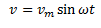From the equation, we deduce that vm is used to signify the amplitude for the oscillating potential to denotes the differences. The angular frequency is given by ω. The current can be calculated by using the Kirchhoff’s loop rule. The equation which forms is as under,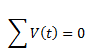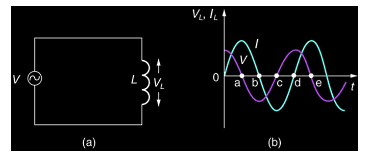Using the above equation in the given circuit, we can write,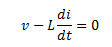Here the second term is the self-induced Faraday emf of the inductor and the L is the term given to the self-inductance of the inductor. The above two equations, that is, the equation for the voltage across the inductor and that derived from the Kirchhoff’s law from the given circuit, give the following equation,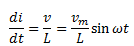Here, from the above equation, we see that the equation for the change in current as a function of time is sinusoidal in nature and is in the same phase as the source voltage and its amplitude is given by Vm/L. We can calculate the current through the inductor by integrating the above quantity with respect to time as: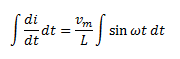The above equation leads us to the value of current as given by,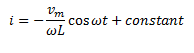The integration constant has the dimension of current and is time-independent. Since the source has an EMF that oscillates symmetrically about zero, the current it sustains also oscillates symmetrically about zero, so that no constant or time-independent component currently exists. Therefore, the integration constant is zero.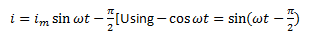Here, the amplitude of the current is given by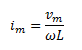The quantity ωL can be said to be equivalent to the resistance of this device and is termed as the inductive resistance. We denote the inductive resistance of the device as XL.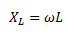Thus, we can say that the amplitude of current in this circuit is given as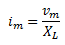Read more: AC voltage source applied across a capacitor
To know more about AC current, electromagnetism and solved problems, click the below video.### Practice Problem

If the inductance of an inductor L = 10 mH and frequency f = 50 KHz. Find the inductive reactance of an inductor?

Solution:

Given L = 10 mH and f = 50 KHz
We know that,
XL = 2πfL
XL = 2×π×80×103×15×10-3
XL = 3.1 KΩ

## Frequently Asked Questions – FAQs

### What is an inductor?

The inductor is a passive two-terminal device that stores energy in a magnetic field when electric current flows through it.

### State Kirchhoff’s Current Law.

Kirchhoff’s Current Law states that “The total current entering a junction or a node is equal to the charge leaving the node as no charge is lost.”

### What is the SI unit of inductive reactance?

The SI unit of inductive reactance is Ohm.

True.

### The number of turns of a solenoid are doubled without changing its length and area of cross section. The self inductance of the solenoid will be increased by how many times?

The self inductance of the solenoid will be increased by 4 times.